# Calculating Geometric Mean Using R and Python

## Recent Posts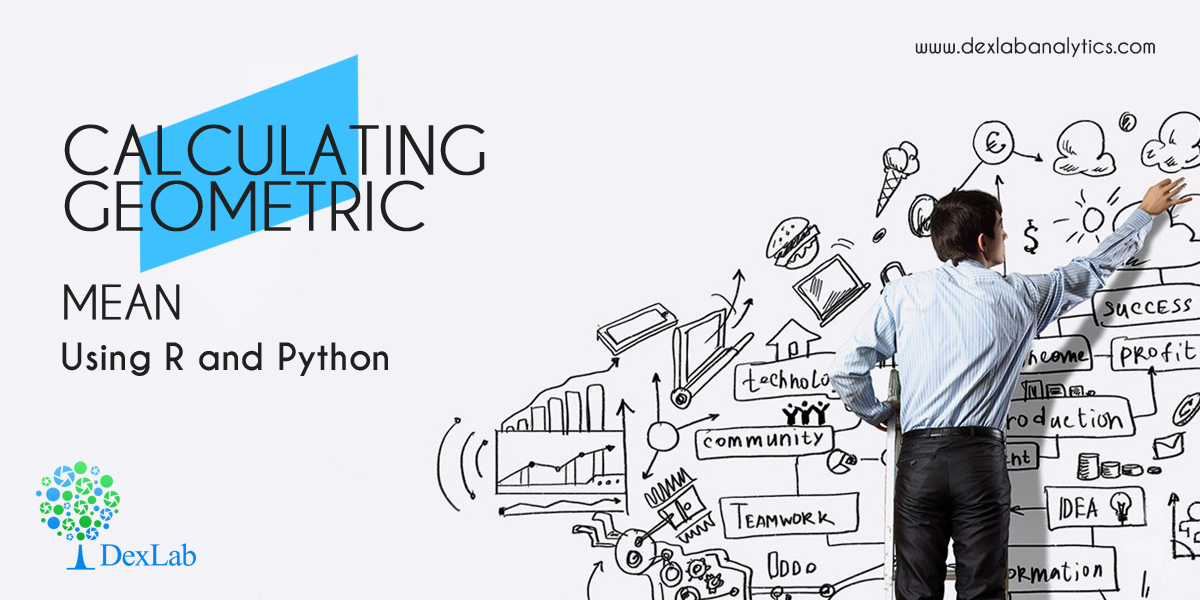In this blog, we are going to discuss the Geometric Mean and its application using Python and R.

Geometric Mean of group of ‘n’ observations is the nth root of their product. It is defined only when all observations have the same sign and none of them is zero.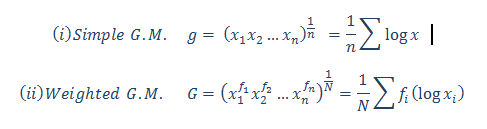#### Calculate the Geometric Mean of the salary increment of 12 employees. From the following table, calculate the average salary increment of the year (2019-2020):-

 Name Salary Increment inPercentage (%) Ritesh 10.09% Heena 15.45% Kritika 9% Anuradha 13.06% Gaurav 20% Prakash 14% Aarti 16% Meena 6.25% Utkarsh 12.85% Chirag 10% Neha 18% Smrita 21.36%

#### Calculate the Geometric Mean in R: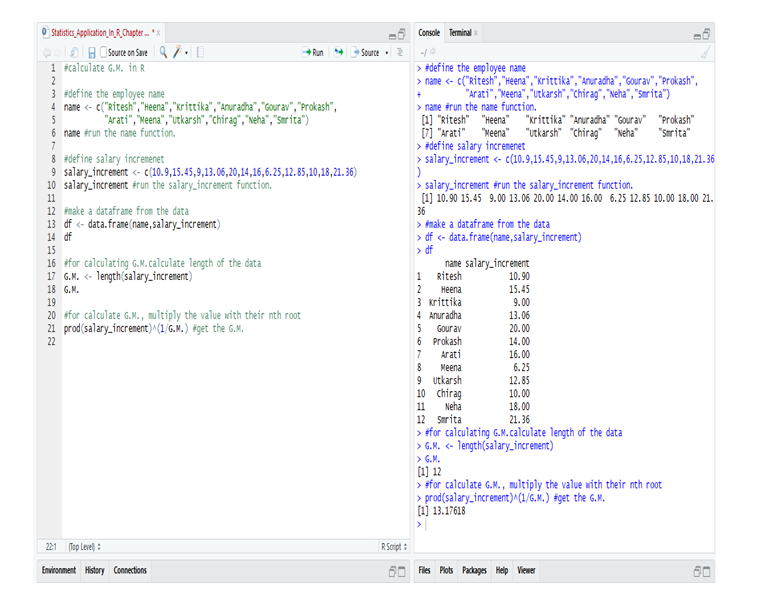So, from the data of the employee’s in R we calculate the G.M. and get that the average salary increment in the year (2019-2020) = 13.17618 or 13.18% (approx).

#### Calculate the Geometric Mean in Python: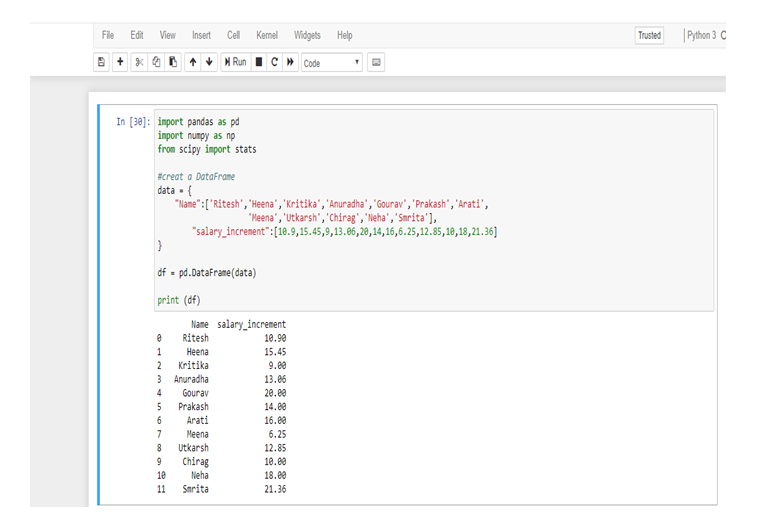First, make a data frame in Python from the following table.

Now, calculate the Geometric Mean from the data-frame.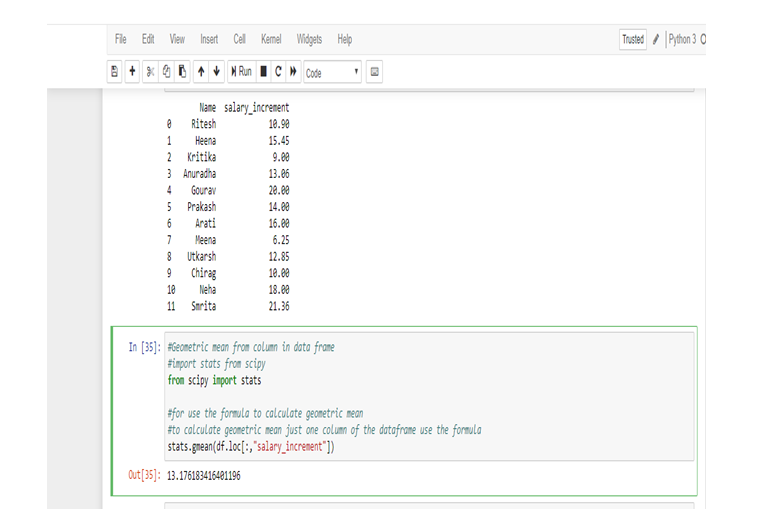So, from the data of the employee’s in Python we calculate the G.M. and get that the average salary increment in the year (2019-2020) = 13.176183416401196 or 13.18% (approx).

We use Geometric Mean for calculating ratios, rates and percentages. And it is not affected by the extreme value or outlier. In this particular problem, we use Geometric Mean because an average of the salary increment of the employee’s not affected by the extreme highest or extreme lowest value, that’s why the salary increment rates of Meena and Smrita do not have any effect on the total average rate.

Geometric Mean gives small value than Arithmetic Mean.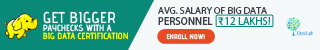Note: This is a continuation of the blog: Statistical Application in R & Python: Chapter 1 – Measure of Central Tendency. It would be better to go through the first installment and then read this one. More blogs are to be followed, so stay tuned.

DexLab Analytics is a premier Python training institute in Delhi. Our industry-relevant courses are carefully crafted by experts. Follow us on Facebook and Instagram.

#### Interested in a career in Data Analyst?### Call us to know more### Gurgaon+91 931 572 5902